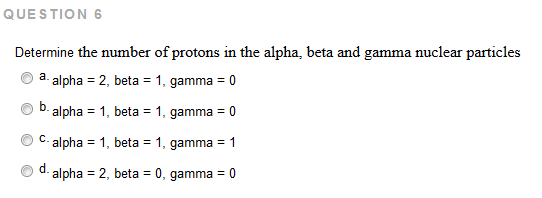# Problem: Determine the number of protons in the alpha, beta and gamma nuclear particlesa. alpha = 2, beta = 1, gamma = 0b. alpha = 1, beta = 1, gamma = 0 c. alpha = 1, beta = 1, gamma = 1d. alpha = 2, beta = 1, gamma = 0

###### FREE Expert Solution
79% (283 ratings)###### Problem Details

Determine the number of protons in the alpha, beta and gamma nuclear particles

a. alpha = 2, beta = 1, gamma = 0

b. alpha = 1, beta = 1, gamma = 0

c. alpha = 1, beta = 1, gamma = 1

d. alpha = 2, beta = 1, gamma = 0